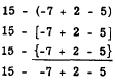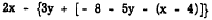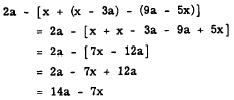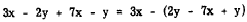Symbols of GroupingCustom SearchSYMBOLS OF GROUPING Often it is desired to group two or more terms to indicate that they are to be considered and treated as though they were one term even though there may be plus and minus signs between them. The symbols of grouping are parentheses ( ) (which we have already used), brackets [ ], braces { }, and the vinculum __. The vinculum is sometimes called the "overscore." The fact that -7 + 2 - 5 is to be subtracted from 15, for example, could be indicated in any one of the following ways:Actually the vinculum is seldom used except in connection with a radical sign, such as inor in a Boolean algebra expression.  Boolean algebra is a specialized kind of symbolic notation which is discussed in Mathematics, Volume 3, NavPers 10073. Parentheses are the most frequently used symbols of grouping. When several symbols are needed to avoid confusion in grouping, parentheses usually comprise the innermost symbols, followed by brackets, and then by braces as the outermost symbols. This arrangement of grouping symbols is illustrated as follows:REMOVING AND INSERTING GROUPING SYMBOLS Discussed in the following paragraphs are various rules governing the removal and ineertion of parentheses, brackets, braces, and the vinculum. Since the rules are the same for all grouping symbols, the discussion in terms of parentheses will serve as a basis for all. Removing Parentheses If parentheses are preceded by a minus sign, the entire quantity enclosed must be regarded as a subtrahend. This means that each term of the quantity in parentheses is subtracted from the expression preceding the minus sign. Accordingly, parentheses preceded by a minus sign can be removed, if the signs of all terms within the parentheses are changed. This may be explained with an arithmetic example. We recall that to subtract one number from another, we change the sign of the subtrahend and proceed as in addition. To subtract -7 from 16, we change the sign of -7 and proceed as in addition, as follows: 16 - (-7) = 16 + 7                = 23 It is sometimes easier to see the result of changing signs in the subtrahend if the minus sign preceding the parentheses is regarded as a multiplier. Thus, the thought process in removing parentheses from an expression such as - (4 - 3 + 2) would be as follows: Minus times plus is �minus, so the first term of the expression with parentheses removed is - 4. (Remember that the 4 in the original expression is understood to be a +4, since it has no sign showing.) Minus times minus is plus, so the second term is +3. Minus times plus is minus, so the third term is -2. The result is - 4 + 3 - 2, which reduces to -3. This same result can be reached just as easily, in an arithmetic expression, by combining the numbers within the parentheses before applying the negative sign which precedes the parentheses. However, in an algebraic expression with no like terms such combination is not possible. The following example shows how the rule for removal of parentheses is applied to algebraic expressions: 2a - (-4x + 3by) = 2a + 4x - 3by Parenthesis preceded by a plus sign can be removed without any other changes, m the following example shows: 2b + (a -b)=2b+a-b-a+b Many expressions contain more than one set of parentheses, brackets, and other symbols of grouping. In removing symbols of grouping, it is possible to proceed from the outside inward or from the inside outward. For the beginner, it is simpler to start on the inside and work toward the outside, collecting terms and simplifying as one proceeds. In the following example the inner grouping symbols are removed first:Enclosing Terms in Parentheses  When it is desired to enclose a group of terms in parentheses, the group of terms remains unchanged if the sign preceding the parentheses is positive. This is illustrated as follows:Note that this agrees with the rule for removing parentheses preceded by a plus sign. If terms are enclosed within parentheses preceded by a minus sign, the signs of all the terms enclosed must be changed as in the following example:Practice problems. In problems 1 .through 4, remove the symbols of grouping and combine like terms. In problems 5 through 8, enclose the first two terms in parentheses preceded by a plus sign (understood) and the last two in parentheses preceded by a minus sign.Integrated Publishing, Inc. - A (SDVOSB) Service Disabled Veteran Owned Small Business